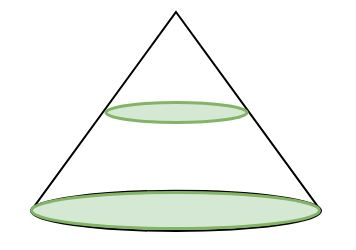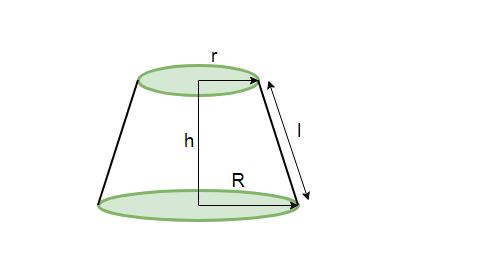# Program for Volume and Surface area of Frustum of Cone

• Last Updated : 23 Apr, 2018

Given slant height, height and radius of a frustum of a cone, we have to calculate the volume and surface area of the frustum of a cone.

Frustum of cone
In geometry, a frustum is the portion of a solid (normally a cone or pyramid) that lies between one or two parallel planes cutting it.
If we cut a right circular cone by a plane parallel to its base, the portion of the solid between this plane and the base is known as the frustum of a cone.

Given below is a right circular cone.The right circular cone after being cut by a plane parallel to its base results in a frustum as follows:which has a circular base at bottom of radius R
circular upper portion with radius r
height h
and slant height l

• Volume of frustum of cone:
• ```Volume (V) = 1/3 * pi * h(r2 + R2 + r*R)

where
r = radius of smaller circle
R = radius of bigger circle (or radius of base of the cone)
h = height of the frustum
```
• Curved Surface Area of frustum of cone:
• ```Curved Surface Area (CSA) = pi * l(R + r)

where
r = radius of smaller circle
R = radius of bigger circle
l = slant height of the frustum
```
• TotalSurface Area of frustum of cone:
• ```Total Surface Area (TSA) = pi * l(R + r) + pi(R2 + r2)

where
r = radius of smaller circle
R = radius of bigger circle
l = slant height of frustum
```

Examples:

```Input : Radius of smaller circle = 3
Radius of bigger circle = 8
Height of frustum = 12
Slant height of frustum = 13
Output :
Volume Of Frustum of Cone : 1218.937
Curved Surface Area Of Frustum of Cone : 449.24738
Total Surface Area Of Frustum of Cone : 678.58344

Input : Radius of smaller circle = 7
Radius of bigger circle = 10
Height of frustum = 4
Slant height of frustum = 5

Output :
Volume Of Frustum of Cone : 917.34436
Curved Surface Area Of Frustum of Cone : 267.03516
Total Surface Area Of Frustum of Cone : 735.1321
```

## C++

 `// CPP program to calculate Volume and``// Surface area of frustum of cone``#include ``using` `namespace` `std;`` ` `float` `pi = 3.14159;`` ` `// Function to calculate Volume of frustum of cone``float` `volume(``float` `r, ``float` `R, ``float` `h)``{``    ``return` `(``float``(1) / ``float``(3)) * pi * h *``                    ``(r * r + R * R + r * R);``}`` ` `// Function to calculate Curved Surface area of``// frustum of cone``float` `curved_surface_area(``float` `r, ``float` `R, ``float` `l)``{``    ``return` `pi * l * (R + r);``}`` ` `// Function to calculate Total Surface area of ``// frustum of cone``float` `total_surface_area(``float` `r, ``float` `R, ``float` `l, ``                                           ``float` `h)``{``    ``return` `pi * l * (R + r) + pi * (r * r + R * R);``}`` ` `// Driver function``int` `main()``{``    ``float` `small_radius = 3;``    ``float` `big_radius = 8;``    ``float` `slant_height = 13;``    ``float` `height = 12;`` ` `    ``// Printing value of volume and surface area``    ``cout << ``"Volume Of Frustum of Cone : "``         ``<< volume(small_radius, big_radius, height) ``         ``<< endl;`` ` `    ``cout << ``"Curved Surface Area Of Frustum of Cone : "``         ``<< curved_surface_area(small_radius, big_radius, ``                                 ``slant_height) << endl;`` ` `    ``cout << ``"Total Surface Area Of Frustum of Cone : "``         ``<< total_surface_area(small_radius, big_radius, ``                                 ``slant_height, height);``    ``return` `0;``}`

## Java

 `// Java program to calculate Volume and Surface area``// of frustum of cone`` ` `public` `class` `demo {`` ` `    ``static` `float` `pi = ``3``.14159f;`` ` `    ``// Function to calculate Volume of frustum of cone``    ``public` `static` `float` `volume(``float` `r, ``float` `R, ``float` `h)``    ``{``        ``return` `(``float``)``1` `/ ``3` `* pi * h * (r * r + R * R +``                                                ``r * R);``    ``}`` ` `    ``// Function to calculate Curved Surface area of``    ``// frustum of cone``    ``public` `static` `float` `curved_surface_area(``float` `r, ``                                   ``float` `R, ``float` `l)``    ``{``        ``return` `pi * l * (R + r);``    ``}`` ` `    ``// Function to calculate Total Surface area of ``    ``// frustum of cone``    ``public` `static` `float` `total_surface_area(``float` `r, ``                         ``float` `R, ``float` `l, ``float` `h)``    ``{``        ``return` `pi * l * (R + r) + pi * (r * r + R * R);``    ``}`` ` `    ``// Driver function``    ``public` `static` `void` `main(String args[])``    ``{``        ``float` `small_radius = ``3``;``        ``float` `big_radius = ``8``;``        ``float` `slant_height = ``13``;``        ``float` `height = ``12``;`` ` `    ``// Printing value of volume and surface area``        ``System.out.print(``"Volume Of Frustum of Cone : "``);``        ``System.out.println(volume(small_radius, ``                            ``big_radius, height));`` ` `        ``System.out.print(``"Curved Surface Area Of"` `+ ``                            ``" Frustum of Cone : "``);``        ``System.out.println(curved_surface_area(small_radius,``                                  ``big_radius, slant_height));``        ``System.out.print(``"Total Surface Area Of"` `+ ``                ``" Frustum of Cone : "``);`` ` `        ``System.out.println(total_surface_area(small_radius, ``                        ``big_radius, slant_height, height));``    ``}``}`

## Python3

 `# Python3 code to calculate ``# Volume and Surface area of``# frustum of cone``import` `math`` ` `pi ``=` `math.pi`` ` `# Function to calculate Volume``# of frustum of cone``def` `volume( r , R , h ):``    ``return` `1` `/``3` `*` `pi ``*` `h ``*` `(r ``            ``*` `r ``+` `R ``*` `R ``+` `r ``*` `R)`` ` `# Function to calculate Curved ``# Surface area of frustum of cone``def` `curved_surface_area( r , R , l ):``    ``return` `pi ``*` `l ``*` `(R ``+` `r)`` ` `# Function to calculate Total  ``# Surface area of frustum of cone``def` `total_surface_area( r , R , l , h ):``    ``return` `pi ``*` `l ``*` `(R ``+` `r) ``+` `pi ``*` `(r``                            ``*` `r ``+` `R ``*` `R)``     ` `# Driver Code``small_radius ``=` `3``big_radius ``=` `8``slant_height ``=` `13``height ``=` `12`` ` `# Printing value of volume ``# and surface area``print``(``"Volume Of Frustum of Cone : "``                                ``,end``=``'')``print``(volume(small_radius, big_radius,``                                ``height))`` ` `print``(``"Curved Surface Area Of Frustum"``+``                    ``" of Cone : "``,end``=``'')``print``(curved_surface_area(small_radius,``                ``big_radius,slant_height))`` ` `print``(``"Total Surface Area Of Frustum"``+``                    ``" of Cone : "``,end``=``'')``print``(total_surface_area(small_radius, ``        ``big_radius,slant_height, height))`` ` `# This code is contributed by "Sharad_Bhardwaj".`

## C#

 `// C# program to calculate Volume and ``// Surface area of frustum of cone``using` `System;`` ` `public` `class` `demo {`` ` `    ``static` `float` `pi = 3.14159f;`` ` `    ``// Function to calculate ``    ``// Volume of frustum of cone``    ``public` `static` `float` `volume(``float` `r, ``float` `R, ``float` `h)``    ``{``        ``return` `(``float``)1 / 3 * pi * h * (r * r + R * ``                                        ``R + r * R);``    ``}`` ` `    ``// Function to calculate Curved``    ``// Surface area of frustum of cone``    ``public` `static` `float` `curved_surface_area(``float` `r, ``                                ``float` `R, ``float` `l)``    ``{``        ``return` `pi * l * (R + r);``    ``}`` ` `    ``// Function to calculate Total``    ``// Surface area of frustum of cone``    ``public` `static` `float` `total_surface_area(``float` `r, ``float` `R, ``                                           ``float` `l, ``float` `h)``    ``{``        ``return` `pi * l * (R + r) + pi *``                    ``(r * r + R * R);``    ``}`` ` `    ``// Driver function``    ``public` `static` `void` `Main()``    ``{``        ``float` `small_radius = 3;``        ``float` `big_radius = 8;``        ``float` `slant_height = 13;``        ``float` `height = 12;`` ` `    ``// Printing value of volume ``    ``// and surface area``    ``Console.Write(``"Volume Of Frustum of Cone : "``);``     ` `    ``Console.WriteLine(volume(small_radius, ``                    ``big_radius, height));`` ` `    ``Console.Write(``"Curved Surface Area Of"` `+ ``                     ``" Frustum of Cone : "``);``                      ` `    ``Console.WriteLine(curved_surface_area(small_radius,``                            ``big_radius, slant_height));``                             ` `    ``Console.Write(``"Total Surface Area Of"` `+ ``                    ``" Frustum of Cone : "``);`` ` `    ``Console.WriteLine(total_surface_area(small_radius, ``                    ``big_radius, slant_height, height));``    ``}``}`` ` `// This article is contributed by vt_m`

## PHP

 ``

Output:

```Volume Of Frustum of Cone : 1218.937
Curved Surface Area Of Frustum of Cone : 449.24738
Total Surface Area Of Frustum of Cone : 678.58344
```

My Personal Notes arrow_drop_up